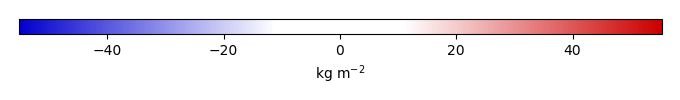# Mean State

Period Mean (original grids) [Pg]
Model Period Mean (intersection) [Pg]
Model Period Mean (complement) [Pg]
Benchmark Period Mean (intersection) [Pg]
Benchmark Period Mean (complement) [Pg]
Bias [kg m-2]
Bias Score 
Spatial Distribution Score 
Overall Score 
Benchmark [-] 76.5
CLM5PHSOFF [-] 67.2 55.6 11.8 76.1 0.362 -7.37 0.569 0.584 0.576
CLM5PHSON [-] 101. 90.3 10.0 76.1 0.362 6.17 0.533 0.297 0.415
Period Mean (original grids) [Pg]
Model Period Mean (intersection) [Pg]
Model Period Mean (complement) [Pg]
Benchmark Period Mean (intersection) [Pg]
Benchmark Period Mean (complement) [Pg]
Bias [kg m-2]
Bias Score 
Spatial Distribution Score 
Overall Score 
Benchmark [-] 113.
CLM5PHSOFF [-] 74.8 35.0 37.8 113. 0.00185 -16.4 0.498 0.534 0.516
CLM5PHSON [-] 133. 70.5 59.6 113. 0.00185 -9.00 0.560 0.825 0.693
Period Mean (original grids) [Pg]
Model Period Mean (intersection) [Pg]
Model Period Mean (complement) [Pg]
Benchmark Period Mean (intersection) [Pg]
Benchmark Period Mean (complement) [Pg]
Bias [kg m-2]
Bias Score 
Spatial Distribution Score 
Overall Score 
Benchmark [-] 12.2
CLM5PHSOFF [-] 63.0 2.73 61.5 12.2 -9.64 0.471 0.627 0.549
CLM5PHSON [-] 91.8 5.31 88.9 12.2 -6.98 0.546 0.822 0.684
Period Mean (original grids) [Pg]
Model Period Mean (intersection) [Pg]
Model Period Mean (complement) [Pg]
Benchmark Period Mean (intersection) [Pg]
Benchmark Period Mean (complement) [Pg]
Bias [kg m-2]
Bias Score 
Spatial Distribution Score 
Overall Score 
Benchmark [-] 97.3
CLM5PHSOFF [-] 87.0 76.4 11.6 97.2 0.0996 -6.59 0.606 0.730 0.668
CLM5PHSON [-] 119. 110. 10.0 97.2 0.0996 4.55 0.545 0.395 0.470
Period Mean (original grids) [Pg]
Model Period Mean (intersection) [Pg]
Model Period Mean (complement) [Pg]
Benchmark Period Mean (intersection) [Pg]
Benchmark Period Mean (complement) [Pg]
Bias [kg m-2]
Bias Score 
Spatial Distribution Score 
Overall Score 
Benchmark [-] 5.12
CLM5PHSOFF [-] 39.5 3.01 35.6 5.12 -7.97 0.610 0.640 0.625
CLM5PHSON [-] 44.2 3.46 40.1 5.12 -6.30 0.638 0.616 0.627
Period Mean (original grids) [Pg]
Model Period Mean (intersection) [Pg]
Model Period Mean (complement) [Pg]
Benchmark Period Mean (intersection) [Pg]
Benchmark Period Mean (complement) [Pg]
Bias [kg m-2]
Bias Score 
Spatial Distribution Score 
Overall Score 
Benchmark [-] 475.
CLM5PHSOFF [-] 886. 275. 611. 474. 1.51 -8.82 0.533 0.389 0.461
CLM5PHSON [-] 1.06e+03 433. 622. 474. 1.51 -1.41 0.534 0.642 0.588
Period Mean (original grids) [Pg]
Model Period Mean (intersection) [Pg]
Model Period Mean (complement) [Pg]
Benchmark Period Mean (intersection) [Pg]
Benchmark Period Mean (complement) [Pg]
Bias [kg m-2]
Bias Score 
Spatial Distribution Score 
Overall Score 
Benchmark [-] 116.
CLM5PHSOFF [-] 73.1 60.2 12.4 116. 0.373 -9.15 0.507 0.720 0.614
CLM5PHSON [-] 123. 115. 7.60 116. 0.373 0.749 0.492 0.623 0.557
Period Mean (original grids) [Pg]
Model Period Mean (intersection) [Pg]
Model Period Mean (complement) [Pg]
Benchmark Period Mean (intersection) [Pg]
Benchmark Period Mean (complement) [Pg]
Bias [kg m-2]
Bias Score 
Spatial Distribution Score 
Overall Score 
Benchmark [-] 0.0131
CLM5PHSOFF [-] 20.3 0.00162 19.6 0.0131 -5.18 0.415 4.03e-22 0.208
CLM5PHSON [-] 32.2 0.0178 31.5 0.0131 2.19 0.692 2.46e-23 0.346
Period Mean (original grids) [Pg]
Model Period Mean (intersection) [Pg]
Model Period Mean (complement) [Pg]
Benchmark Period Mean (intersection) [Pg]
Benchmark Period Mean (complement) [Pg]
Bias [kg m-2]
Bias Score 
Spatial Distribution Score 
Overall Score 
Benchmark [-] 46.1
CLM5PHSOFF [-] 45.7 21.6 24.5 46.1 0.00725 -11.9 0.511 0.316 0.413
CLM5PHSON [-] 75.2 36.0 39.5 46.1 0.00725 -4.90 0.560 0.579 0.570
Period Mean (original grids) [Pg]
Model Period Mean (intersection) [Pg]
Model Period Mean (complement) [Pg]
Benchmark Period Mean (intersection) [Pg]
Benchmark Period Mean (complement) [Pg]
Bias [kg m-2]
Bias Score 
Spatial Distribution Score 
Overall Score 
Benchmark [-] 0.232
CLM5PHSOFF [-] 7.06 0.0855 7.54 0.232 -3.26 0.442 0.475 0.459
CLM5PHSON [-] 11.5 0.289 11.9 0.232 0.143 0.533 0.808 0.671

# Temporally integrated period mean

BENCHMARK MEANMODEL MEANBIASBIAS SCORESPATIAL TAYLOR DIAGRAMMODEL COLORS# Spatially integrated regional mean

MODEL COLORS# All Models

BenchmarkCLM5PHSOFFCLM5PHSON# Data Information

Approach: I just read the data from the original data file and converted the latitude arrange from original 90N->90S to 90S->90N. The unit was convert from original 0.1 KgC/m2 to KgC/m2

Temporal resolution: yearly

General information: This product was derived from Northern Circumpolar Soil Carbon Database V2.2 (NCSCDv22) in top 1m.

Spatial resolution: 0.5x0.5 degree